﻿

# UNIT 29: Planes of Symmetry

Planes of Symmetry can be used to section a symmetrical casting model, so that only a portion of the model needs to be meshed and simulated. Why do this? The reason is so that a simulation can be run with fewer nodes and thus in a shorter time, but still get the same resolution in terms of node size.

For example, if a casting is symmetrical in halves, it is possible to cut the model in half with a plane of symmetry. Running a simulation with 500,000 nodes on one half of the model would produce the same results as running a simulation of the full model with 1,000,000 nodes. The amount of time to run the smaller simulation would be significantly shorter.

As an example, consider the following model of an idler casting. This model is exactly symmetrical down the middle: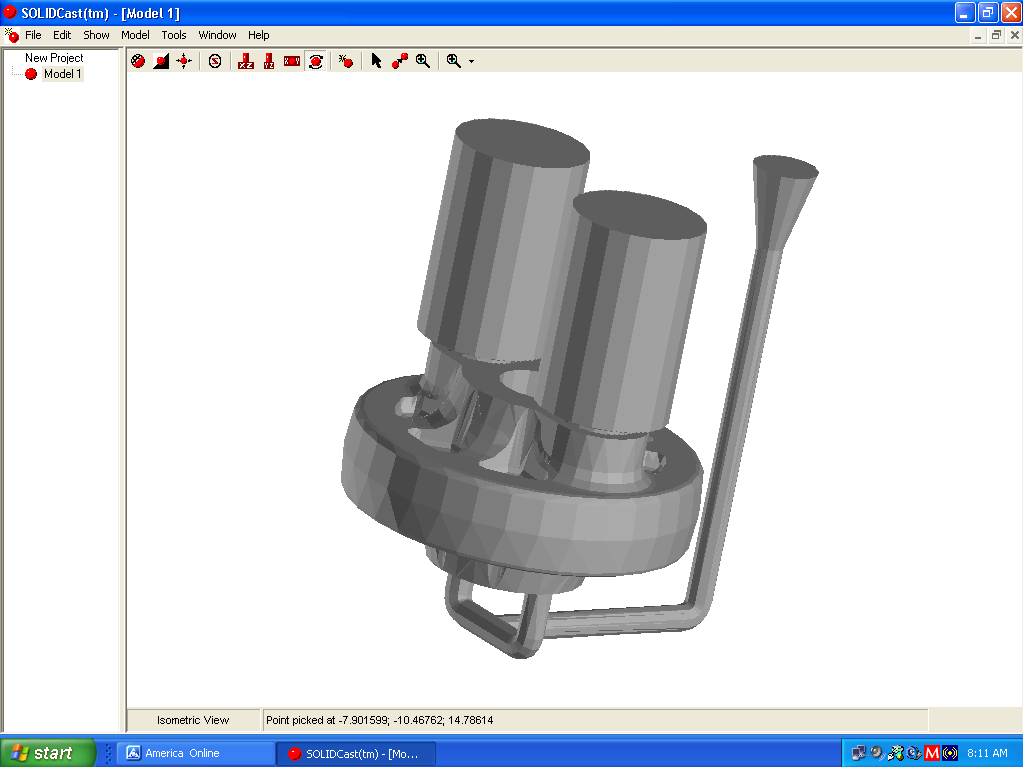In this case, we could place a plane of symmetry along the X-axis of the part. This model was conveniently created with the center of the casting at the origin point of space (where X and Y are 0) so it is easy to set up a plane of symmetry here.

To create the plane of symmetry, select Model…Options… and click on the Planes of Symmetry tab. You will see the following window appear: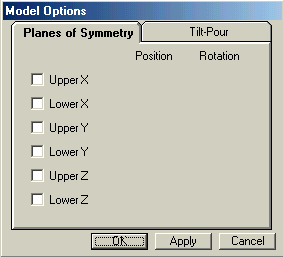In this case, we want a lower-Y Plane of Symmetry at the position Y=0.000. Therefore, click on the small square to the left of Lower Y to place a check mark there. The window will then appear as follows: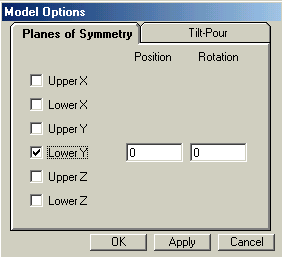Now click the button labeled Apply. This places the Plane of Symmetry at Y=0. If you wanted to place the plane at a different location, just enter a different number in the field under Position. Also, it is possible to rotate an X or Y Plane of Symmetry to make, for example, a wedge shape from a round casting.

The Plane of Symmetry will appear on the model as follows: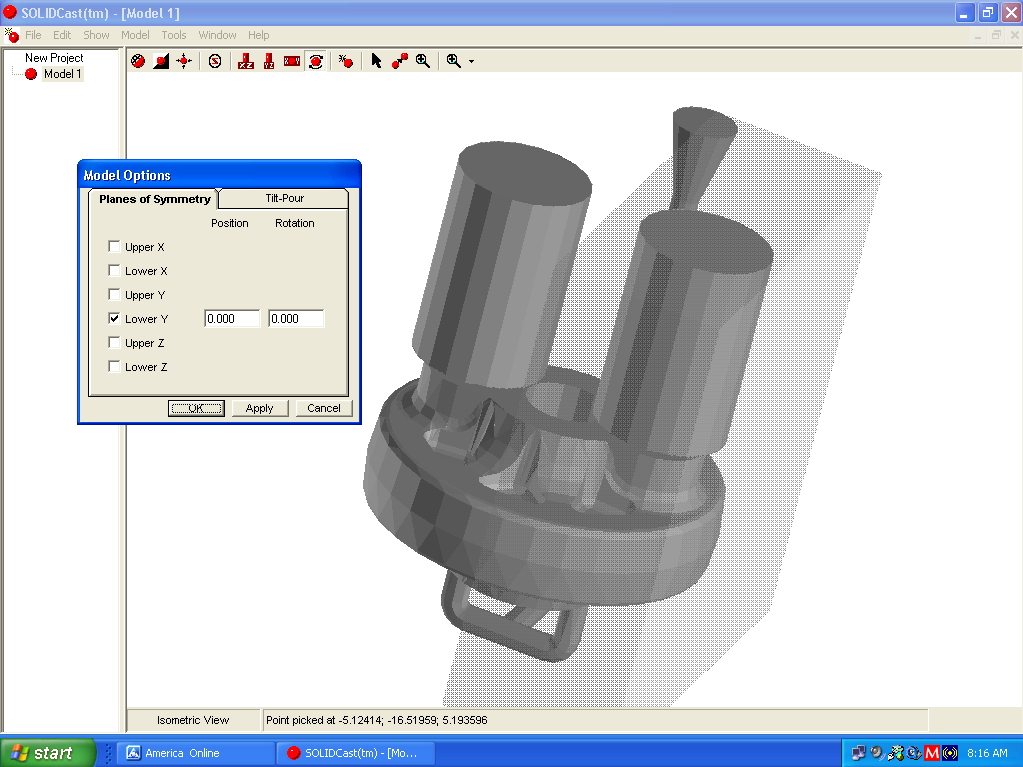The Plane of Symmetry is a rectangular block that covers half the model. This indicates that when the model is meshed, only one-half will actually appear in the mesh. This can be verified by meshing the model and viewing the result: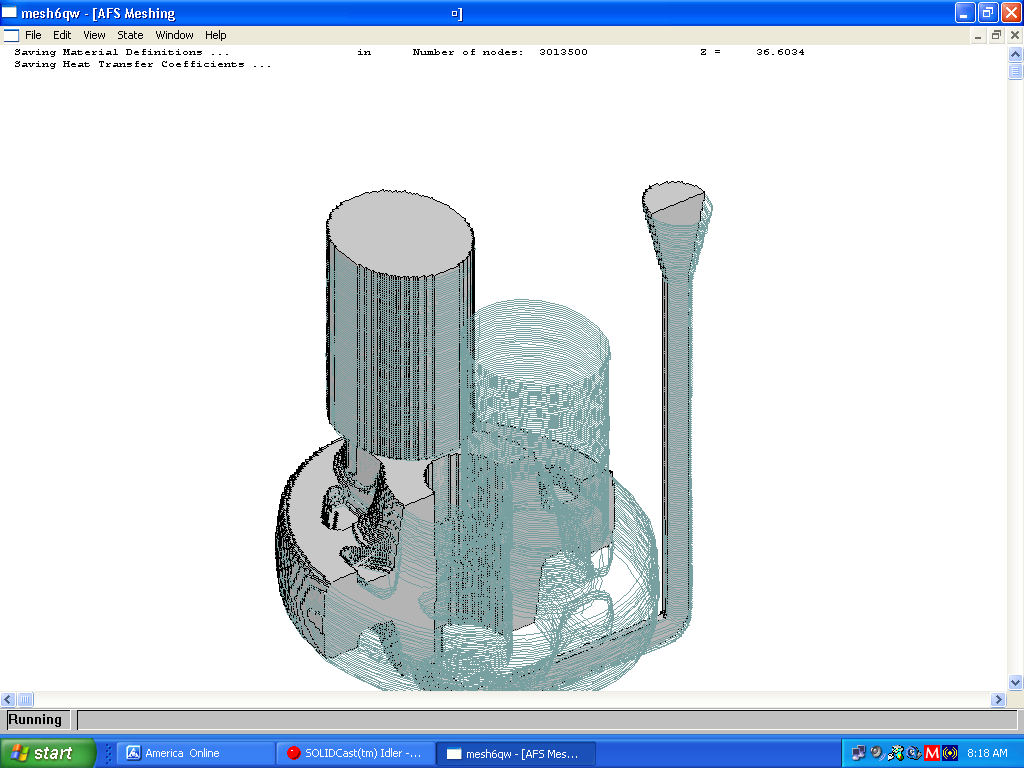Here the meshing operation meshes only one-half the casting. The other half of the casting appears in outline (because this was an imported STL shape), but only the meshed half will actually be run in the simulation. After simulation, the data can be mirrored and displayed on the full casting model.